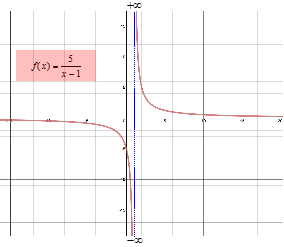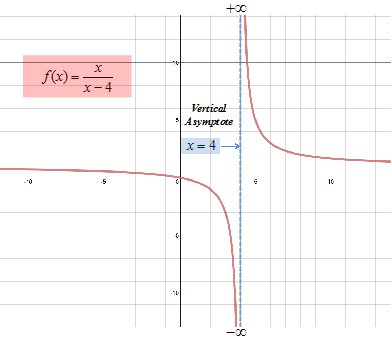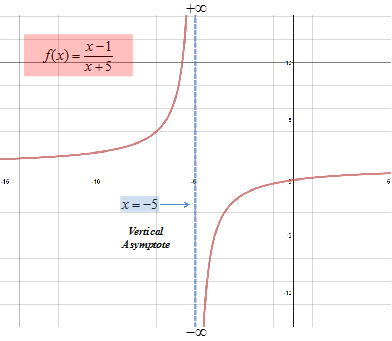# Limits: Infinite Limits

To discuss infinite limits, let's investiagte the funtion $f\left(x\right)=\frac{5}{x-1}$. Looking at the graph of this function shown here, you can see that as $x\to {1}^{-}$ the value of f(x) decreases without bound and when $x\to {1}^{+}$ the value of f(x) increases without bound. A table of values will show the same behavior.

 x $f\left(x\right)=\frac{5}{x-1}$ x $f\left(x\right)=\frac{5}{x-1}$ 0.9 -50 1.00001 500,000 0.99 -500 1.0001 50,000 0.999 -5000 1.001 5000 0.9999 -50,000 1.01 500 0.99999 -500,000 1.1 50A limit in which f(x) increases or decreases without bound as the value of x approaches an arbitrary number c is called an infinite limit.

This does not mean that a limit exists or that $\infty$ is a number. In fact the limit does not exist. The values of $±\infty$ simply tell how the limit fails to exist because the values as x approaches c increase/decrease without bound.

Infinite limits are denoted by:

$\underset{x\to a}{\mathrm{lim}}f\left(x\right)=\infty$

and is read "the limit of f(x) as x approaches a is infinity".

DEFINITION OF AN INFINITE LIMIT

Let f(x) be a function that can be defined on either side of a point a, and may or may not be defined at a:

$\underset{x\to a}{\mathrm{lim}}f\left(x\right)=+\infty$      or      $\underset{x\to a}{\mathrm{lim}}f\left(x\right)=-\infty$

means as x approaches a, but not equal to a, the value of f(x) increase/decreases without bound.

The line at which the limit of a function increases or decreases without bound is called a vertical asymptote.

DEFINITION OF A VERTICAL ASYMPTOTE

The line x = a is a vertical asymptote of f(x) if one of the following is true:

$\underset{x\to a}{\mathrm{lim}}f\left(x\right)=\infty$      $\underset{x\to {a}^{-}}{\mathrm{lim}}f\left(x\right)=\infty$      $\underset{x\to {a}^{+}}{\mathrm{lim}}f\left(x\right)=\infty$

$\underset{x\to a}{\mathrm{lim}}f\left(x\right)=-\infty$     $\underset{x\to {a}^{-}}{\mathrm{lim}}f\left(x\right)=-\infty$     $\underset{x\to {a}^{+}}{\mathrm{lim}}f\left(x\right)=-\infty$

Let's find the infinite limits in a couple examples.

Example 1: Find the $\underset{x\to {4}^{+}}{\mathrm{lim}}\frac{x}{x-4}$ and $\underset{x\to {4}^{-}}{\mathrm{lim}}\frac{x}{x-4}$, sketch the graph and define the vertical asymptote.

Step 1: Find the $\underset{x\to {4}^{+}}{\mathrm{lim}}\frac{x}{x-4}$

Create a table of values for f(x) as $x\to {4}^{+}$ or justify the behavior of the values for f(x). Do not include x = 4.

 x $\frac{x}{x-4}$ 4.1 41 4.01 401 4.001 4001 4.0001 40,001

As values for x get closer and closer to 4 but remain larger than 4, the denominator becomes a smaller and smaller positive number so the quotient becomes increasingly larger without bound.

$\underset{x\to {4}^{+}}{\mathrm{lim}}\frac{x}{x-4}=\infty$

Step 2: Find the $\underset{x\to {4}^{-}}{\mathrm{lim}}\frac{x}{x-4}$

Create a table of values for f(x) as $x\to {4}^{-}$ or justify the behavior of the values for f(x). Do not include x = 4.

 x $\frac{x}{x-4}$ 3.9 -39 3.99 -399 3.999 -3999 3.9999 39,999

As values for x get closer and closer to 4 but remain smaller than 4, the denominator becomes a smaller and smaller negative number so the quotient becomes increasingly large negative number without bound.

$\underset{x\to {4}^{-}}{\mathrm{lim}}\frac{x}{x-4}=-\infty$

Step 3: Sketch the graph.Step 4: Define the vertical asymptote.

Because $\underset{x\to {4}^{-}}{\mathrm{lim}}f\left(x\right)=-\infty$ ; $\underset{x\to {4}^{+}}{\mathrm{lim}}f\left(x\right)=\infty$, the line $x=4$ is a vertical asymptote.

Example 2: Find the $\underset{x\to -5}{\mathrm{lim}}\frac{x-1}{x+5}$ if it exists. If it does not exist explain why.

Step 1: Find the $\underset{x\to -{5}^{+}}{\mathrm{lim}}\frac{x-1}{x+5}$

Create a table of values for f(x) as $x\to -5$ or justify the behavior of the values for f(x). Do not include x = -5.

 x $\frac{x-1}{x+5}$ -4.9 -59 -4.99 -599 -4.999 -5999 -4.9999 -59,999

As values for x get closer and closer to -5 but remain larger than -5, the denominator becomes a smaller and smaller positive number while the numerator approaches -6 so the quotient becomes increasingly negative without bound.

$\underset{x\to {5}^{+}}{\mathrm{lim}}\frac{x-1}{x+5}=-\infty$

Step 2: Find the $\underset{x\to -{5}^{-}}{\mathrm{lim}}\frac{x-1}{x+5}$

Create a table of values for f(x) as $x\to -{5}^{-}$ or justify the behavior of the values for f(x). Do not include x = -5.

 x $\frac{x-1}{x+5}$ -5.1 61 -5.01 601 -5.001 6001 -5.0001 60,001

As values for x get closer and closer to -5 but remain smaller than -5, the denominator becomes a smaller and smaller negative number while the numerator approaches -6 so the quotient becomes an increasingly larger positive number without bound.

$\underset{x\to -{5}^{-}}{\mathrm{lim}}\frac{x-1}{x+5}=\infty$

Step 3: Sketch the graph.Step 4: Determine if the function has a limit.

Because $\underset{x\to -{5}^{-}}{\mathrm{lim}}f\left(x\right)=\infty$ ; $\underset{x\to -{5}^{+}}{\mathrm{lim}}f\left(x\right)=-\infty$, the line $x=-5$ is a vertical asymptote. Because the limits increase without bound, no limit exists. Remember, an infinite limit is not a limit but merely states how the limit fails.

 Related Links: Math algebra Limits: Limit Laws General Differentiation Rules Calculus Topics

To link to this Limits: Infinite Limits page, copy the following code to your site: